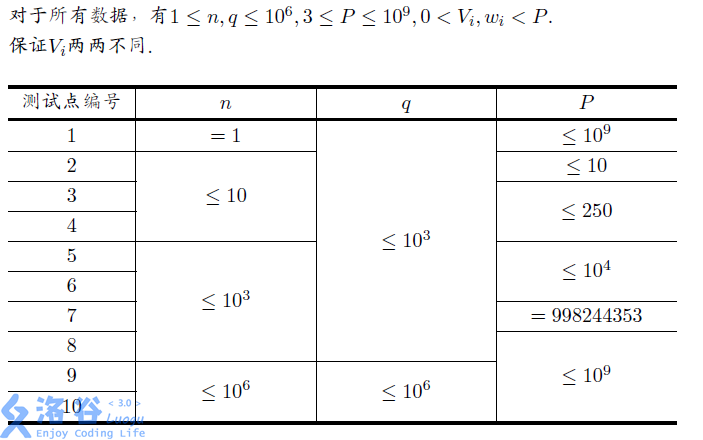P4495 [HAOI2018]奇怪的背包

• 218通过
• 702提交
• 题目提供者 CCF_NOI
• 评测方式 云端评测
• 标签 动态规划,动规,dp 枚举,暴力 概率论,统计 各省省选 2018 河南 O2优化
• 难度 省选/NOI-
• 时空限制 2000ms / 512MB
• 提示：收藏到任务计划后，可在首页查看。

题目描述

小$C$非常擅长背包问题，他有一个奇怪的背包，这个背包有一个参数$P$，当他 向这个背包内放入若干个物品后，背包的重量是物品总体积对$P$取模后的结果． 现在小$C$有$n$种体积不同的物品，第$i$种占用体积为$V_i$，每种物品都有无限个． 他会进行$q$次询问，每次询问给出重量$w_i$，你需要回答有多少种放入物品的方 案，能将一个初始为空的背包的重量变为$w_i$．注意，两种方案被认为是不同的， 当且仅当放入物品的种类不同，而与每种物品放入的个数无关．不难发现总的方 案数为$2^n$. 由于答案可能很大，你只需要输出答案对1e9+7取模的结果．

输入输出格式

输入格式：

从文件$knapsack.in$中读入数据. 第一行三个整数$n$,$q$,$P$，含义见问题描述. 接下来一行$n$个整数表示$V_i$. 接下来一行$q$个整数表示$w_i$.

输出格式：

输出到文件$knapsack.out$中. 输出$q$行，每行一个整数表示答案．

输入输出样例

输入样例#1： 复制
3 3 6
1 3 4
5 2 3
输出样例#1： 复制
5
6
6

说明HAOI2018 round1 T1

提示
标程仅供做题后或实在无思路时参考。
请自觉、自律地使用该功能并请对自己的学习负责。
如果发现恶意抄袭标程，将按照I类违反进行处理。